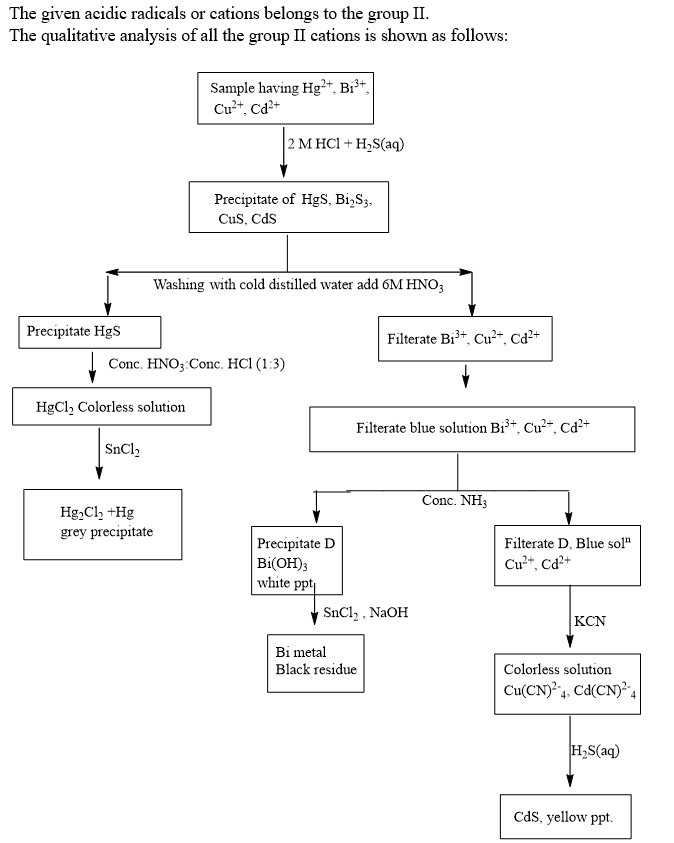# Question & Answer: Experiment 37 Qualitative Analysis of Group II Cations Precipitation and Separation of Group…..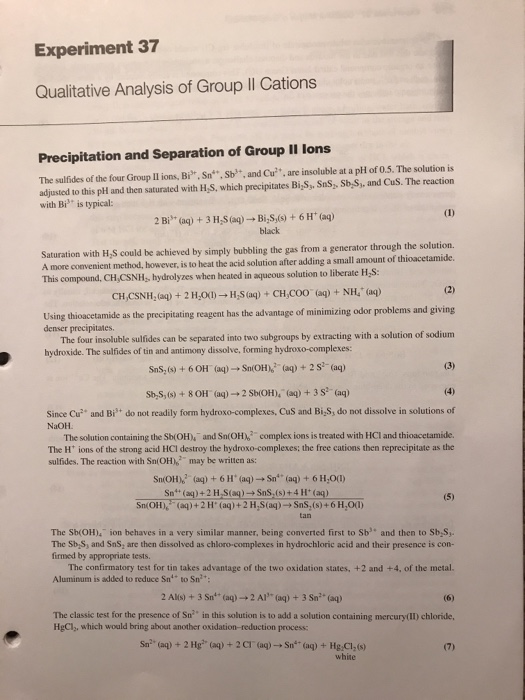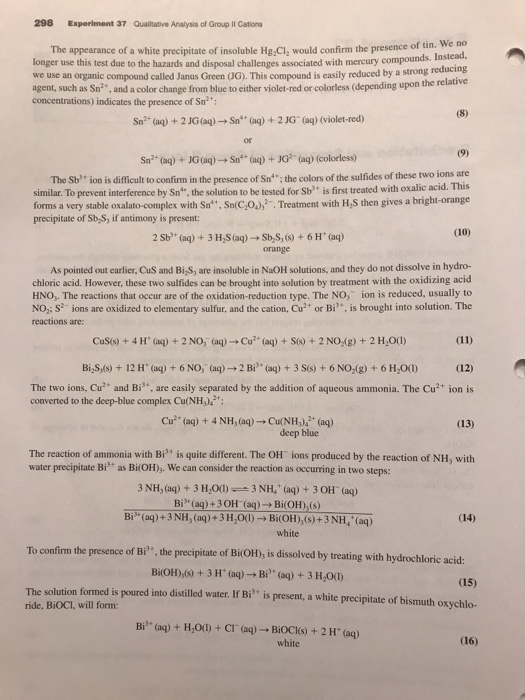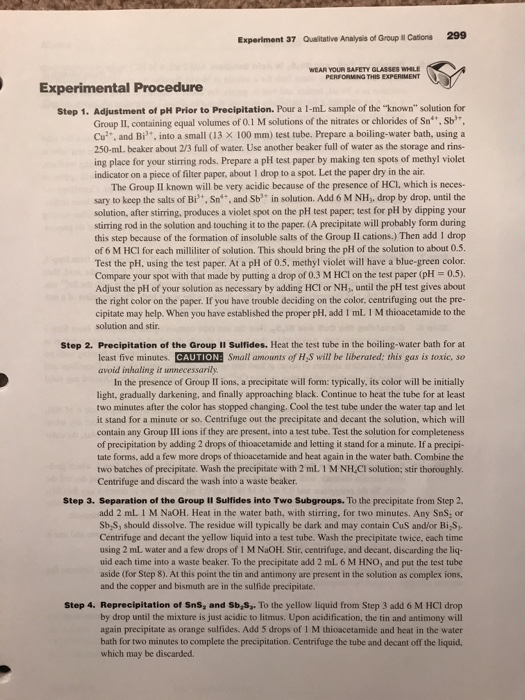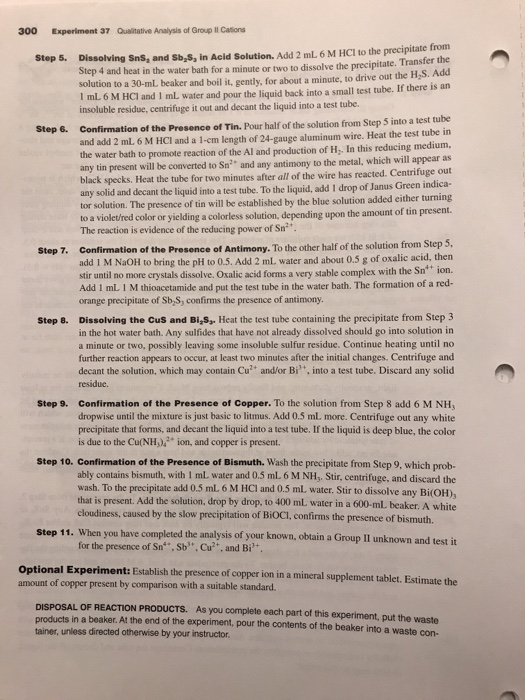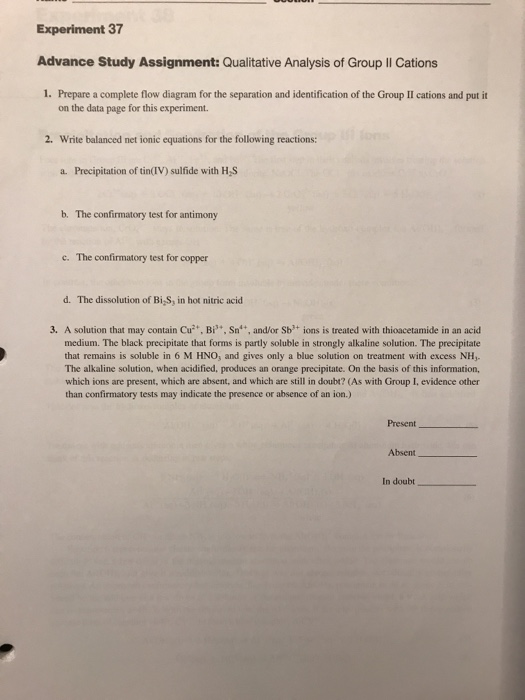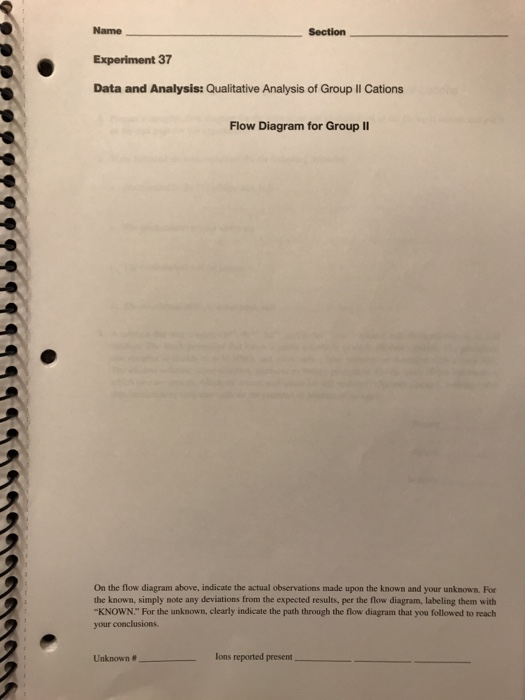Experiment 37 Qualitative Analysis of Group II Cations Precipitation and Separation of Group II lons The sulfides of the four Group II ions, Bi, Sn Sb, and Cu'”, are insoluble at a pH of 0.5. The solution is adjusted to this plt and then saturated with H,S, which precipitates Bi,S, Sns, Sb,S, and Cus. The reaction with Bi” is typical: 2 Bis+ (aq) + 3 H,s(aq) ? Bizs,(s) + 6 H+ (aq) black Saturation with H,S could be achieved by simply bubbling the gas from a generator through the solution. A more convenicnt method, however, is to heat the acid solution after adding a small amount of thioacetamide This compound, CHCSNH;, hydrolyzes when heated in aqueous solution to liberate H,S CH,CSNH,(aq) + 2 H,01)? H,s(aq) + CH,COO. (aq) + NH4+(aq) as the precipitating reagent has the advantage of minimizing odor problems and giving denser precipitates. The four insoluble sulfides can be separated hydroxide. The sulfides of tin and antimony dissolve, forming hydroxo-complexes: into two subgroups by extracting with a solution of sodium Sas, (s) + 6 OH’ (aq) ? Sn(OHA:-(aq) + 2 s-(aq) St,S, (s) + 8 OH. (aq) ? 2 Sb(OH)’ (aq) + 3 S2-(aq) Since Cu” and Bi NaOH do not readily form hydroxo-complexes, CuS and Bi S, do not dissolve in solutions of complex ions is treated with HCI and thioacetamide. The solution containing the Sb(OH), and Sn(OH) The H” ions of the strong acid HCI destroy the hydroxo-complexes; the free cations then reprecipitate as the Sn(OH)”aq) + 6 H’aq) ? sn’, (aq) + 6 H,O(1) SnOH)–(aq)+ 2 H’ (aq) +2H2S(aq)? SnS:(s) + 6H,01) The Sb(OH). ion behaves in a very similar manner, being converted first to Sb and then to Sb S, sulfides. The reaction with Sn(OH) may be written as: The Sb S, and SnS, are then dissolved as chloro-complexes in hydrochloric acid and their presence is con- firmed by appropriate tests. The confirmatory test for tin takes advantage of the two oxidation states, +2 and +4, of the metal. Aluminum is added to reduce Sn to Sn The classic test for the presence of Sn2″ in this solution is to add a solution containing mercury(I) chloride, HgCl, which would bring about another oxidation-reduction process: white